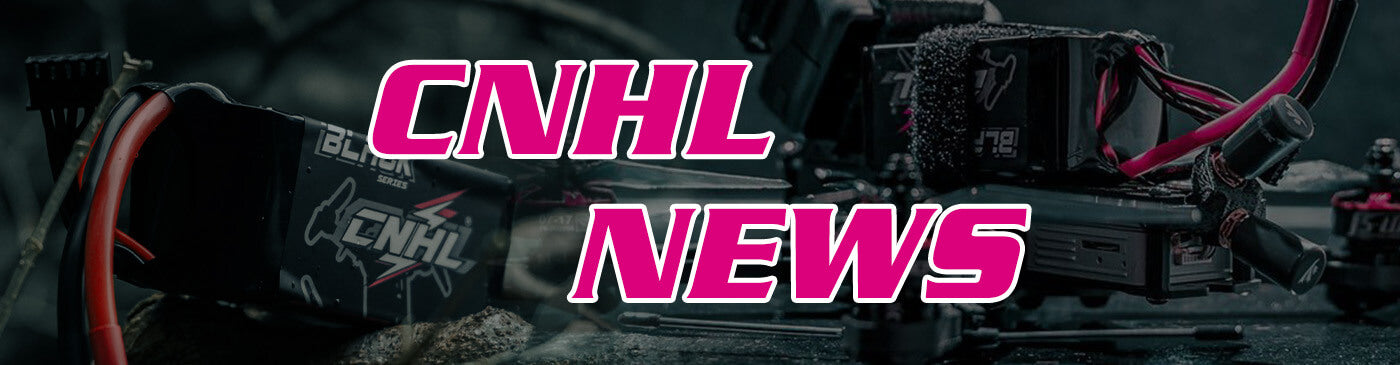Extra US\$40 OFF
On orders US\$150+ | Promo Code: CNHL40
Extra US\$40 OFF
On orders US\$150+ | Promo Code: CNHL40# What are the modeling types of electrical characteristics of 3s lipo battery?

The modeling of 3s lipo battery is divided into mechanism modeling, experimental modeling and hybrid modeling according to the modeling methods. The above models are within the range of the 3s lipo battery electrical characteristics model. The electrical characteristic models of 3s lipo battery are generally divided into three categories: black box model, electrochemical model and equivalent circuit model. Next, CNHL will briefly introduce the above three types of models.

## 3s lipo battery black box model

The black box model is a linear or nonlinear function that describes the voltage response characteristics of the 3s lipo battery. It shifts the focus from the internal mechanism of the 3s lipo battery to the data itself, which determines that it can be very flexible to establish the model structure and parameterize , but lacks the essential physical meaning. The difficulty of black-box modeling is that model performance is sensitive to the quantity and quality of training data, and usually requires data-driven algorithms, such as neural networks and support vector regression.

## 3s lipo battery electrochemical model

The electrochemical model of 3s lipo battery is realized by analyzing the internal reaction mechanism of 3s lipo battery and establishing partial differential equations of electrode and electrolyte kinetics. While accurately predicting macroscopic physical parameters, it can also simulate the distribution of important microscopic physical quantities inside the 3s lipo battery, so it is more suitable for the optimization design and safety analysis of the 3s lipo battery. However, the complexity of the electrochemical model is high, and the actual system computing power may not meet the theoretical requirements, so the current research mainly focuses on how to simplify the model so that it can be applied to the 3s lipo battery management technology.

## 3s lipo battery equivalent circuit model

The 3s lipo battery equivalent circuit model (ECM) uses circuit elements such as capacitors, resistors and constant voltage sources to form a circuit network to simulate the dynamic voltage response characteristics of the 3s lipo battery. The relationship between the parameters in this type of model is direct and obvious, and generally contains a relatively small number, which makes the mathematical description of the state space easier, so it is widely used in system simulation and practical management. 3s lipo batteryECM contains a variety of model structure frameworks. The advantage of a simple combined structure is to achieve a fast and low-cost model, but the simulation accuracy will also be lower, and a model with a more comprehensive combined structure will improve the accuracy and also bring Greater complexity increases the difficulty of identifying parameters and calculation process.

Therefore, in practice, the advantages and disadvantages of the existing equivalent circuit models should be weighed, and a suitable circuit model should be selected through comprehensive analysis. Several related concepts are briefly described below. Ohmic internal resistance: It is composed of electrode material, electrolyte, internal resistance of diaphragm and contact resistance of various parts. Polarization internal resistance: the resistance caused by the polarization reaction phenomenon during the electrochemical reaction, including the resistance caused by electrochemical polarization and concentration difference, and the polarization capacitor is connected in parallel to form an RC loop, which is used to simulate the polarization of 3s lipo battery generation and greatly reduce the dynamic characteristics exhibited in the process.

The above is the whole content of the electrical characteristics modeling type of 3s lipo battery brought to you by CNHL. The electrical characteristics models of 3s lipo battery are generally divided into three categories: black box model, electrochemical model and equivalent circuit model; black box model is a A linear or nonlinear function that describes the voltage response characteristics of 3s lipo battery, which shifts the focus from the internal mechanism of 3s lipo battery to the data itself; the electrochemical model of 3s lipo battery is established by analyzing the internal reaction mechanism of 3s lipo battery. The 3s lipo battery equivalent circuit model (ECM) uses circuit elements such as capacitors, resistors and constant voltage sources to form a circuit network to simulate the dynamic voltage response characteristics of the 3s lipo battery.